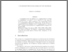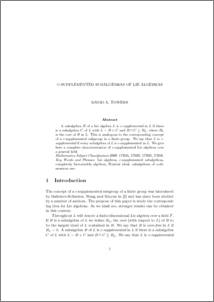# C-Supplemented Subalgebras of Lie Algebras.

Towers, David A. (2008) C-Supplemented Subalgebras of Lie Algebras. Journal of Lie Theory, 18 (3). pp. 717-724.Preview
PDF (c-supplemented_lie_algebras.pdf)
c-supplemented_lie_algebras.pdf

A subalgebra $B$ of a Lie algebra $L$ is c-{\it supplemented} in $L$ if there is a subalgebra $C$ of $L$ with $L = B + C$ and $B \cap C \leq B_L$, where $B_L$ is the core of $B$ in $L$. This is analogous to the corresponding concept of a c-supplemented subgroup in a finite group. We say that $L$ is c-{\it supplemented} if every subalgebra of $L$ is c-supplemented in $L$. We give here a complete characterisation of c-supplemented Lie algebras over a general field.View Item